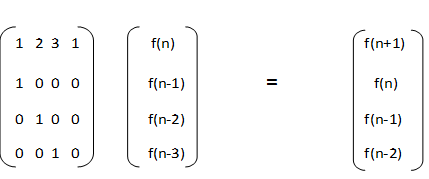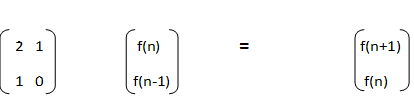Saturday, February 23, 2013

Fibonacci Series Part II

In the last post we discussed about evaluating the nth Fibonacci number in logarithmic time O(logn).

Lets us look at another problem on the similar lines.
Given a series
F(n) = F(n-1) + 2*F(n-2) + 3*F(n-3) + F(n-4)

Lets try to solve the problem in the similar fashion,

The matrix multiplication looks like below,The running time to compute the nth term of the series will be
(sizeof(M) ^3) * log(n) = 64*log(n)

Can we optimize it further ?

Lets again go over the series once more
F(n) = F(n-1) + 2*F(n-2) + 3*F(n-3) + F(n-4)
F(n-1) = F(n-2) + 2*F(n-3) + 3*F(n-4) + F(n-5)
F(n) - F(n-1) = F(n-1) + F(n-2) + F(n-3)  - 2*F(n-4) - F(n-5)
After some more rearrangement it reduces to
F(n) = 2* F(n-1) + F(n-2)
Some of you must have recognized it as Pell's number.

So the matrix multiplication reduces toSo the final runtime reduces to O(8* log(n))